## Channel Estimation

The LTE Toolbox™ product uses orthogonal frequency division multiplexing (OFDM) as its digital multicarrier modulation scheme. Channel estimation plays an important part in an OFDM system. It is used for increasing the capacity of orthogonal frequency division multiple access (OFDMA) systems by improving the system performance in terms of bit error rate.

To facilitate the estimation of the channel characteristics, LTE uses cell-specific reference signals (pilot symbols) inserted in both time and frequency. These pilot symbols provide an estimate of the channel at given locations within a subframe. Through interpolation, it is possible to estimate the channel across an arbitrary number of subframes.

### Channel Estimation Overview

The pilot symbols in LTE are assigned positions within a subframe depending on the eNodeB cell identification number and which transmit antenna is being used, as shown in the following figure.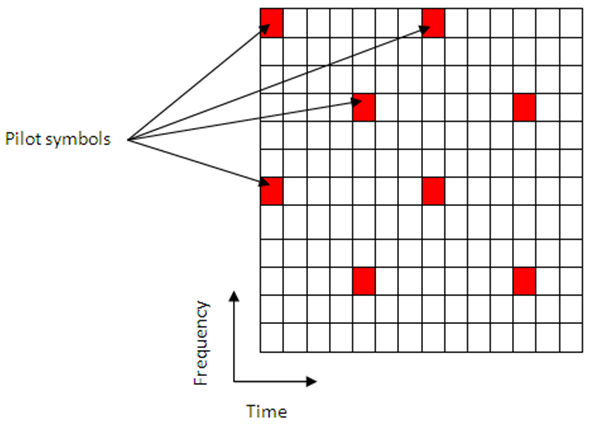The unique positioning of the pilots ensures that they do not interfere with one another and can be used to provide a reliable estimate of the complex gains imparted onto each resource element within the transmitted grid by the propagation channel.

Both transmit and receive chains and the propagation channel model are shown in the following block diagram.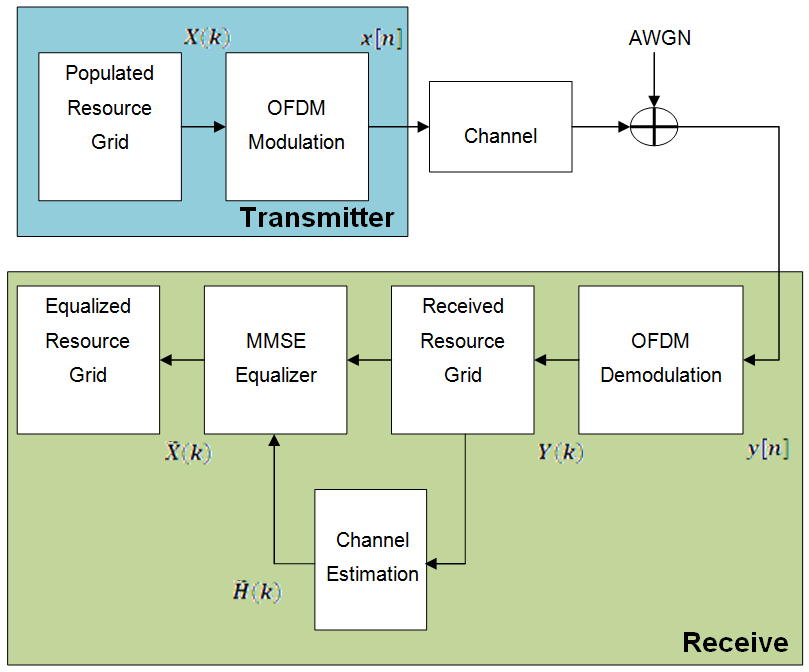The populated resource grid represents several subframes containing data. This grid is then OFDM modulated and passed through the model of the propagation channel. Channel noise in the form of additive white Gaussian noise (AWGN) is added before the signal enters the receiver. Once inside the receiver the signal is OFDM demodulated and a received resource grid can be constructed. The received resource grid contains the transmitted resource elements which have been affected by the complex channel gains and the channel noise. Using the known pilot symbols to estimate the channel, it is possible to equalize the effects of the channel and reduce the noise on the received resource grid.

LTE assigns each antenna port a unique set of locations within a subframe to which to map reference signals. Because no other antenna transmits data at these locations in time and frequency, channel estimation for multi-antenna configurations can be performed. The channel estimation algorithm extracts the reference signals for a transmit/receive antenna pair from the received grid. The least squares estimates of the channel frequency response at the pilot symbols are calculated as described in On Channel Estimation in OFDM Systems . The least squares estimates are then averaged to reduce any unwanted noise from the pilot symbols. Because it is possible that no pilots are located near the subframe edge, virtual pilot symbols are created to aid the interpolation process near the edge of the subframe. Using the averaged pilot symbol estimates and the calculated virtual pilot symbols, interpolation is then carried out to estimate the entire subframe. This process is demonstrated in the following block diagram.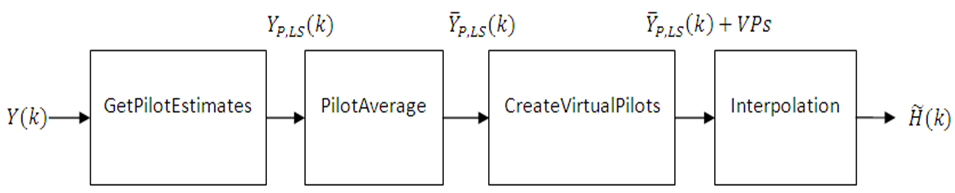### Get Pilot Estimates Subsystem

The first step in determining the least squares estimate is to extract the pilot symbols from their known location within the received subframe. Because the value of these pilot symbols is known, the channel response at these locations can be determined using the least squares estimate. The least squares estimate is obtained by dividing the received pilot symbols by their expected value.

`$Y\left(k\right)=H\left(k\right)X\left(k\right)+noise$`

Where:

• $Y\left(k\right)$ is a received complex symbol value.

• $X\left(k\right)$ is a transmitted complex symbol value.

• $H\left(k\right)$ is a complex channel gain experienced by a symbol.

Known pilot symbols can be sent to estimate the channel for a subset of REs within a subframe. In particular, if pilot symbol ${X}_{P}\left(k\right)$ is sent in an RE, an instantaneous channel estimate ${\stackrel{˜}{H}}_{P}\left(k\right)$ for that RE can be computed using:

`${\stackrel{˜}{H}}_{P}\left(k\right)=\frac{{Y}_{P}\left(k\right)}{{X}_{P}\left(k\right)}={H}_{P}\left(k\right)+noise$`

Where:

• ${Y}_{P}\left(k\right)$ represents the received pilot symbol values.

• ${X}_{P}\left(k\right)$ represents the known transmitted pilot symbol values.

• ${\stackrel{˜}{H}}_{P}\left(k\right)$ is the true channel response for the RE occupied by the pilot symbol.

### Pilot Average Subsystem

To minimize the effects of noise on the channel estimates, the least square estimates are averaged using an averaging window. This simple method produces a substantial reduction in the level of noise found on the pilot REs. The following two pilot symbol averaging methods are available.

• `'TestEVM'` — follows the method described in TS 36.141 , Annex F.3.4.

• `'UserDefined'` — allows you to define the window size and direction of averaging used on the pilot symbols plus other settings used for the interpolation.

#### `'TestEVM'`

The first method, `'TestEVM'`, uses the approach described in TS 36.141 , Annex F.3.4. Time averaging is performed across each subcarrier that contains a pilot symbol, resulting in a column vector containing an average amplitude and phase for each subcarrier that is carrying a reference signal.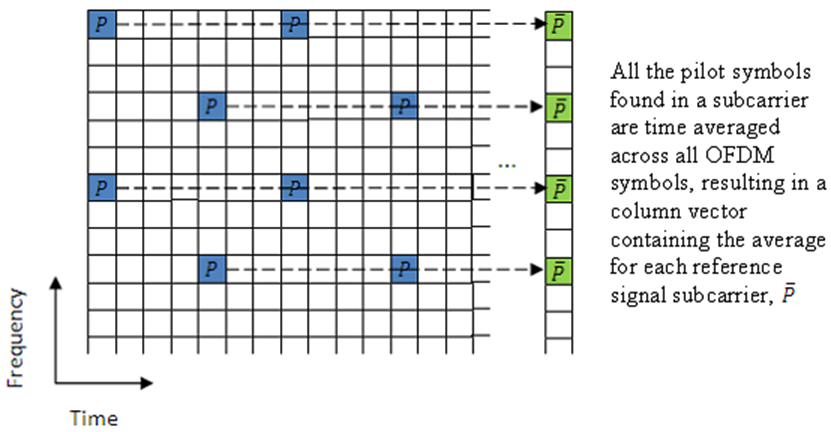The averages of the pilot symbol subcarriers are then frequency averaged using a moving window of maximum size 19.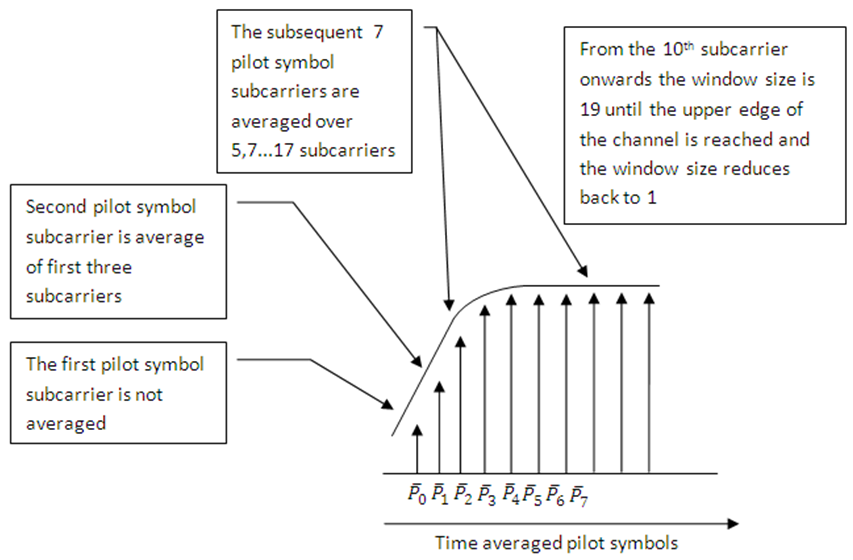### Note

When using `'TestEVM'` pilot symbol averaging, there are no user-defined parameters and control of channel estimation parameters is not possible. The estimation is performed using the method described in TS 36.141 . Except that averaging across 10 subframes is not strictly required. The `lteDLChannelEstimate` function averages across the number of subframes included in the input `rxgrid`. The greater the number of subframes in `rxgrid`, the more effective the noise averaging in the time direction.

#### `'UserDefined'`

The second pilot symbol averaging method, `'UserDefined'`, allows the user to define the size of the averaging window, which direction averaging will be done in (time, frequency or both) and certain aspects of the interpolation that can be adjusted to suit the available data. For more information, see Interpolation Subsystem.

The averaging window size is defined in terms of resource elements. Any pilot symbols located within the window are used to average the value of the pilot symbol found at the centre of the window. The window size must be an odd number ensuring that there is a pilot at the center.

### Note

Averaging the channel estimates at pilot symbol locations is a simple yet powerful tool, but the window size must be carefully chosen. Using a large window size on a fast fading channel could result in averaging out not only noise, but also channel characteristics. Performing too much averaging on a system with a small amount of noise can have an adverse effect on the quality of the channel estimates. Therefore, using a large averaging window for a fast changing channel could cause the estimate of the channel to appear flat, resulting in a poor estimate of the channel and affecting the quality of the equalization.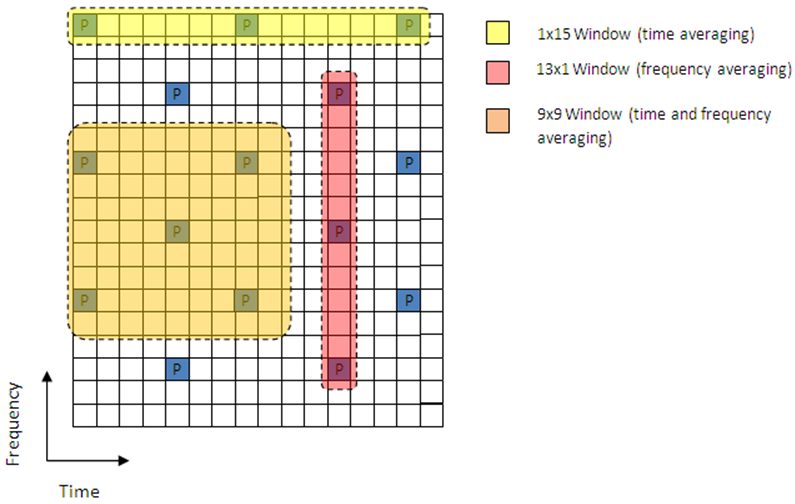### Create Virtual Pilots Subsystem

In many instances, edges of the resource grid do not contain any pilot symbols. This effect is shown in the resource grid in the following figure.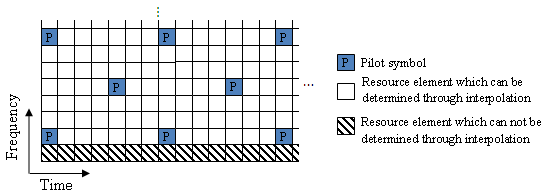In this case, channel estimates at the edges cannot be interpolated from the pilot symbols. To overcome this problem, virtual pilot symbols are created. The function `lteDLChannelEstimate` creates virtual pilot symbols on all the edges of the received grid to allow cubic interpolation.

#### Virtual Pilot Placement

Virtual pilot symbols are created as shown in the following figure.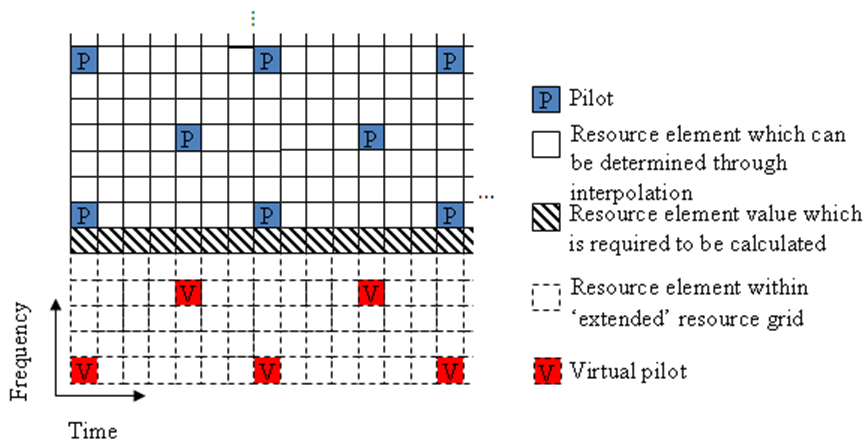In this system, the resource grid is extended, with virtual pilot symbols created in locations which follow the original reference signal pattern. The presence of virtual pilot symbols allows the channel estimate at the resource elements, which previously could not be calculated by interpolation, to be calculated by interpolation using the original and virtual pilot symbols.

#### Calculating Virtual Pilot Symbol Values

The virtual pilot symbols are calculated using the original pilot symbols. For each virtual pilot symbol, the value is calculated following these steps:

1. The closest 10 ordinary pilots in terms of Euclidian distance in time and frequency are selected. The search is optimized to consider 10 these pilots, rather than checking all possible pilots. Based on the possible configurations of the cell RS, using 10 pilots provides sufficient time and frequency diversity in the pilots for the virtual pilot calculation.

2. Using this set of the 10 pilots, the closest three pilot symbols are selected. These three symbols must occupy at least two unique subcarriers and two unique OFDM symbols.

3. Using this set of three pilots, two vectors are created. One vector between the closest and furthest pilot symbols, and one vector between the second closest and furthest pilot symbols.

4. The cross-product of these two vectors is calculated to create a plane on which the three points reside.

5. The plane is extended to the position of the virtual pilot to calculate the value based on one of the actual pilot values.

This diagram shows the virtual pilot calculation.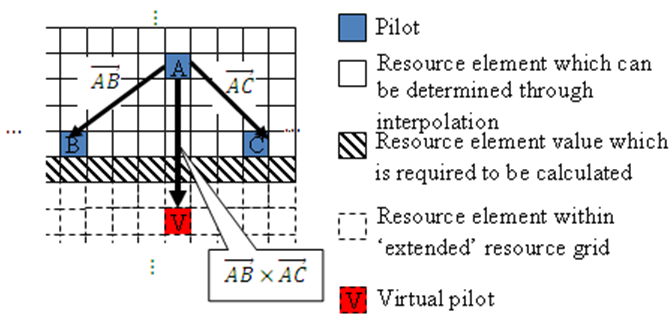### Note

Virtual pilots are only created for the MATLAB® `'linear'` and `'cubic'` interpolation methods.

### Interpolation Subsystem

Once the noise has been reduced or removed from the least squares pilot symbol averages and sufficient virtual pilots have been determined, it is possible to use interpolation to estimate the missing values from the channel estimation grid. The `lteDLChannelEstimate` function has two pilot symbol averaging methods, `'TestEVM'` and `'UserDefined'`. The pilot symbol averaging methods also define the interpolation method performed to obtain the channel estimate.

The `'TestEVM'` pilot averaging method described in TS 36.141 , Annex F.3.4, requires the use of simple linear interpolation on the time-averaged and frequency-averaged column vector. The interpolation is one-dimensional, since it only estimates the values between the averaged pilot symbol subcarriers in the column vector. The resulting vector is then replicated and used as the channel estimate for the entire resource grid.

The `'UserDefined'` pilot-averaging method performs two-dimensional interpolation to estimate the channel response between the available pilot symbols. An interpolation window is used to specify which data is used to perform the interpolation. The `InterpWindow` field defines the causal nature of the available data. Valid settings for `cec`.`InterpWindow` are `'Causal'`, `'Non-causal'`, or `'Centered'`.

Use the `InterpWindow` setting:

• `'Causal'` when using past data.

• `'Non-causal'` when using future data. It is the opposite of `'Causal'`. Relying only on future data is commonly referred to as an anti-causal method of interpolation.

• `'Centered'` or `'Centred'` when using a combination of past, present, and future data.

The size of this interpolation window can also be adjusted to suit the available data. To specify this window size, set the `InterpWinSize` field.

### Noise Estimation

The performance of some receivers can be improved through knowledge of the noise power present on the received signal. The function `lteDLChannelEstimate` provides an estimate of the noise power spectral density (PSD) using the estimated channel response at known reference signal locations. The noise power can be determined by analyzing the noisy least squares estimates and the noise averaged estimates.

The noisy least-squares estimates from the Get Pilot Estimates Subsystem and the noise averaged pilot symbol estimates from the Pilot Average Subsystem provide an indication of the channel noise. The least-squares estimates and the averaged estimates contain the same data, apart from additive noise. Simply taking the difference between the two estimates results in a noise level value for the least squares channel estimates at pilot symbol locations. Considering again,

`${\stackrel{˜}{H}}_{P}\left(k\right)=\frac{{Y}_{P}\left(k\right)}{{X}_{P}\left(k\right)}={H}_{P}\left(k\right)+noise$`

Averaging the instantaneous channel estimates over the smoothing window, we have

`${\stackrel{˜}{H}}_{P}^{AVG}\left(k\right)=\frac{1}{|S|}\sum _{m\in S}{\stackrel{˜}{H}}_{P}\left(m\right)\approx {H}_{P}\left(k\right)$`

where S is the set of pilots in the smoothing window and |S| is the number of pilots in S. Thus, an estimate of the noise at a particular pilot RE can be formed using:

`$\stackrel{˜}{noise}={\stackrel{˜}{H}}_{P}\left(m\right)-{\stackrel{˜}{H}}_{P}^{AVG}\left(k\right)$`

In practice, it is not possible to remove all the noise using averaging. Because it is only possible to reduce the noise, only an estimate of the noise power can be made.

### Note

In the case of a noise free system or system with a high SNR, averaging could have a detrimental effect on the quality of the least squares estimates.

Using the value of the noise power found in the channel response at pilot symbol locations, the noise power per resource element (RE) can be calculated by taking the variance of the resulting noise vector. The noise power per RE for each transmit and receive antenna pair is calculated and stored. The mean of this matrix is returned as the estimate of the noise power per RE.

For a demonstration on how to set up a full transmit and receive chain for channel estimation, see PDSCH Transmit Diversity Throughput Simulation. In this example, multiple antennas are used and transmission is simulated through a propagation channel model.

 3GPP TS 36.141. “Evolved Universal Terrestrial Radio Access (E-UTRA); Base Station (BS) Conformance Testing.” 3rd Generation Partnership Project; Technical Specification Group Radio Access Network. URL: https://www.3gpp.org.

 Van de Beek, J.-J., O. Edfors, M. Sandell, S. K. Wilson, and P. O. Borjesson. “On Channel Estimation in OFDM Systems." Vehicular Technology Conference, IEEE 45th, Volume 2, IEEE, 1995.